Copyright (C) 2012-present, The Computational Data Science Lab
https://github.com/cdslaborg/paramonte
References

# Sampling a 4-dimensional MultiVariate Normal distribution (MVN) via the ParaMonte library's ParaDRAM routine

NOTE
If you are viewing an HTML version of this MATLAB live script on the web, you can download the corresponding MATLAB live script *.mlx file to this HTML page at,
This code, along with other MATLAB live scripts, is located at,
https://github.com/cdslaborg/paramontex/tree/main/MATLAB/mlx
Once you download the file, open it in MATLAB to view and interact with its contents, which is the same as what you see on this page.
NOTE
This ParaDRAM sampler example is also available in Python language as a Jupyter Notebook, on this page,
This Jupyter Notebook, along with other Python Jupyter Notebooks, is located at,
https://github.com/cdslaborg/paramontex/tree/main/Python/Jupyter
IMPORTANT - macOS users
If you are a macOS (Darwin) user and you have downloaded the prebuilt ParaMonte libraries from the GitHub release page to use on your macOS system, read this troubleshooting page before continuing.

## The logic of Monte Carlo sampling is very simple

==========================================================
Suppose we have a mathematical objective function defined on an ndim-dimensional domain. Typically, this domain is defined on the space of real numbers. In general, understanding and visualizing the structure of such objective functions is an extremely difficult task, if not impossible. As a better alternative, you employ Monte Carlo techniques that can randomly explore the structure of the objective function and find the function's extrema.
In the following example, one of the simplest such objective functions, the Multivariate Normal Distribution (MVN), is constructed and sampled using the ParaMonte library samplers, here, the ParaDRAM sampler (Delayed-Rejection Adaptive Metropolis-Hastings Markov Chain Monte Carlo sampler).
Suppose we want to sample random points from a MultiVariate Normal (MVN) Probability Density Function (PDF). The PDF equation of MVN is the following,

## Setting up the path to the ParaMonte::MATLAB library

================================================================
First, we will clean up the MATLAB environment and make sure the path to the ParaMonte library is in MATLAB's path list.
clc;
clear all;
close all;
format compact; format long;
%%%%%%%%%%%% IMPORTANT %%%%%%%%%%%%%
% Set the path to the ParaMonte library:
% Change the following path to the ParaMonte library root directory,
% otherwise, make sure the path to the ParaMonte library is already added
% to MATLAB's path list.
pmlibRootDir = './';
%%%%%%%%%%%%%%%%%%%%%%%%%%%%%%%%%%%%
% change MATLAB's working directory to the folder containing this script
% if MATLAB Live Scripts did not create a temporary folder, we would not
% have all of these problems!
try
setwdFilePath = websave("setwd.m","https://github.com/cdslaborg/paramontex/raw/main/MATLAB/mlx/setwd.m");
run(setwdFilePath); % This is a MATLAB script that you can download from the same GitHub location given in the above.
catch % alas, we will have to run the simulations in MATLAB Live Script's temporary folder
filePath = mfilename('fullpath');
[currentDir,fileName,fileExt] = fileparts(filePath); cd(currentDir);
cd(fileparts(mfilename('fullpath'))); % Change working directory to source code directory.
end

## Constructing the objective function

==========================================
The following MATLAB function getLogFunc() returns the natural logarithm of the Probability Density Function (PDF) of the MultiVariate Normal (MVN) distribution,
NDIM = 4; % the number of dimensions of the domain of the objective function
MEAN = [-10; 15.; 20.; 0.0]; % This is the mean of the multivariate normal (MVN) distribution. THIS IS A COLUMN VECTOR.
COVMAT = [ 1.0,.45,-.3,0.0 ...
; .45,1.0,0.3,-.2 ...
; -.3,0.3,1.0,0.6 ...
; 0.0,-.2,0.6,1.0 ...
]; % This is the covariance matrix of the MVN distribution.
INVCOV = inv(COVMAT); % This is the inverse of the covariance matrix of the MVN distribution.
MVN_COEF = NDIM * log( 1. / sqrt(2.*pi) ) + log( sqrt(det(INVCOV)) ); % This is the log of the coefficient used in the definition of the MVN.
getLogFunc = @(point) MVN_COEF - 0.5 * dot( (MEAN-point)' , INVCOV*(MEAN-point) ); % return the logarithm of the objective function: log(MVN)
NOTE
Since the mathematical objective functions (e.g., probability density functions) can take extremely small or large values, we often work with their natural logarithms instead. This is the reason behind the naming convention used in the ParaMonte library for the user's objective functions: getLogFunc, implying that the user must provide a function that returns the natural logarithm of the target objective function.
See MATLAB Live Script for an in-depth discussion of why we need to work with the logarithm of mathematical objective functions in optimization and sampling problems.
IMPORTANT
Note that in MATLAB all vectors and arrays are, by default, column-major, and so is the input value, point, to getLogFunc(). This means that the size of point is (ndim,1). Thus, all other vectors that interact with point, for example, the mean vector, must be also of the same size as point: (ndim,1).

## Running a ParaDRAM simulation on a single processor

==================================================================
We will sample random points from this objective function by calling the ParaDRAM sampler (Parallel Delayed-Rejection Adaptive Metropolis-Hastings Markov Chain Monte Carlo sampler) of the ParaMonte library.
Note: To run the ParaMonte samplers in parallel, visit the ParaMonte library's documentation website.
We will first create an instance of the paramonte class,
pm = paramonte();

### Checking the ParaMonte library's version

==========================================
Before runnnig any simulations, it is good to check which version of the ParaMonte library you have and are working with,
pm.version.interface.get() % get the ParaMonte::MATLAB (interface) version
ans = "ParaMonte MATLAB Interface Version 2.3.0"
pm.version.interface.dump()
ans = "2.3.0"
pm.version.kernel.get() % the ParaMonte::Kernel version (the version of the ParaDRAM sampler)
ans = "ParaMonte MATLAB Kernel Version 1.5.0"
pm.version.kernel.dump()
ans = "1.5.0"
We can also verify the existence of the essential components of the current installation of the ParaMonte library on the system,
pm.verify();
::::::::::::::::::::::::::::::::::::::::::::::::::::::::::::::::::::::::::::::::::::::::::::::: :::: :::: _/_/_/_/ _/_/ _/_/ _/ _/ _/_/_/_/_/ _/ _/ _/ _/_/_/_/ _/ /_/_/ _/_/_/_/ _/ _/ _/ _/_/ _/_/_/ _/_/_/ _/_/_/ _/_/_/ _/ _/ _/_/ _/ _/ _/ _/ _/ _/ _/ _/ _/ _/_/_/_/ _/ _/ _/ _/ _/ _/ _/ _/ _/ _/ _/ _/ _/ _/ _/_/_/ _/_/_/_/ _/ _/_/_/_/ _/_/_/ _/_/_/ _/_/ _/ _/ _/_/ _/_/_/ ParaMonte plain powerful parallel Monte Carlo library Version 2.3.0 :::: :::: ::::::::::::::::::::::::::::::::::::::::::::::::::::::::::::::::::::::::::::::::::::::::::::::: ParaMonte - NOTE: Intel MPI library for 64-bit architecture detected at: ParaMonte - NOTE: ParaMonte - NOTE: "C:\Program Files (x86)\IntelSWTools\compilers_and_libraries_2020.1.216\windows\mpi\intel64\bin" ParaMonte - NOTE: ParaMonte - NOTE: To perform ParaMonte simulations in parallel on a single node, run the ParaMonte - NOTE: following two commands, in the form and order specified, on a MATLAB-aware ParaMonte - NOTE: mpiexec-aware command-line interface, such as the Intel Parallel Studio's command-prompt: ParaMonte - NOTE: ParaMonte - NOTE: "C:\Program Files (x86)\IntelSWTools\compilers_and_libraries_2020.1.216\windows\mpi\intel64\bin\mpivars.bat" ParaMonte - NOTE: ParaMonte - NOTE: mpiexec -localonly -n 3 matlab -batch "main_mpi" ParaMonte - NOTE: ParaMonte - NOTE: where, ParaMonte - NOTE: ParaMonte - NOTE: 0. the first command defines the essential environment variables and, ParaMonte - NOTE: the second command runs in the simulation in parallel, in which, ParaMonte - NOTE: 1. you should replace the default number 3 with the number of ParaMonte - NOTE: processors you wish to assign to your simulation task, ParaMonte - NOTE: 2. main_mpi.m is the MATLAB file which serves as the entry point to ParaMonte - NOTE: your simulation, where you call the ParaMonte sampler routines. ParaMonte - NOTE: ATTN: Notice the MATLAB filename must appear without the file extension. ParaMonte - NOTE: ATTN: Notice the MATLAB filename must be enclosed with quotation marks. ParaMonte - NOTE: 3. -localonly indicates a parallel simulation on only a single node (this ParaMonte - NOTE: flag will obviate the need for MPI library credentials registration). ParaMonte - NOTE: For more information, visit: ParaMonte - NOTE: ParaMonte - NOTE: https://software.intel.com/en-us/get-started-with-mpi-for-windows ParaMonte - NOTE: ParaMonte - NOTE: Note that the above two commands must be executed on a command-line that recognizes ParaMonte - NOTE: both MATLAB and mpiexec applications, such as the Intel Parallel Studio's command-prompt. ParaMonte - NOTE: For more information, in particular, on how to register to run Hydra services ParaMonte - NOTE: for multi-node simulations on Windows servers, visit: ParaMonte - NOTE: ParaMonte - NOTE: https://www.cdslab.org/paramonte ParaMonte - NOTE: To check for the ParaMonte or the MPI library installations status or, ParaMonte - NOTE: to display the above messages in the future, use the following ParaMonte - NOTE: commands on the MATLAB command-line: ParaMonte - NOTE: ParaMonte - NOTE: pm = paramonte(); ParaMonte - NOTE: pm.verify();

### Setting up the ParaDRAM simulation specifications

====================================================
Now, we create an instance of the ParaDRAM sampler class,
The simplest scenario is to run the simulation with the default specifications that are appropriately determined by the ParaDRAM sampler. However, for the purpose of clarity reproducibility, we will specify a few simulation specs,
For a complete list of all ParaDRAM simulation specifications, visit the ParaDRAM simulation specifications webpage.

#### The simulation output file names and storage path

We will store all output files in a directory with the same name as this MATLAB Live Script's name.
By default, all ParaDRAM simulation output files are named properly (see this page for a review of ParaDRAM simulation output files). In general, it is a good habit to specify an output file prefix for any ParaDRAM simulation. This way, the restart of an incomplete simulation, if it happens for some reason, would be seamless and doable by just rerunning the simulation, without any change of any sort to any parts of the codes or the simulation.
We will prefix all simulation output files the same as the filename of this script,
pmpd.spec.outputFileName = "./out/mvn_serial";
pmpd.spec.helpme("outputFileName");
The above outputFileName will result in the generation of a folder named ./out/, which will keep the simulation output files, all of which are prefixed with mvn_serial.
NOTE
If the specified prefix ends with a forward slash / on Linux/macOS or with a backslash \ on Windows, then the prefix provided will serve as the directory in which the output files will be stored.

#### Ensuring the reproducibility of the results

We will also initialize the random seed to a fixed number to ensure the reproducibility of the simulation results in the future,
pmpd.spec.randomSeed = 3751;
pmpd.spec.helpme("randomSeed");
For the sake of illustration, let's also ask for a modest refinement (decorrelation) of the final sample,
pmpd.spec.sampleRefinementCount = 1;
pmpd.spec.helpme("sampleRefinementCount");

#### Setting the output sample size to a non-default value

Not so important, but we will also specify a chainSize here, 50000, the number of unique points to be sampled from the objective function. This number is smaller than the default 100,000 unique sample points of the sampler.
pmpd.spec.chainSize = 50000;
pmpd.spec.helpme("chainSize");

#### Requesting an ASCII-formatted output restart file (for illustration purposes)

For the sake of illustration, we will also request an ASCII-format restart output file,
pmpd.spec.restartFileFormat = "ascii";
pmpd.spec.helpme("restartFileFormat");
Note, however, that you do not want to request an ACSII-formatted output restart file in your real production simulation as it will slow down your simulation. Just do not set this property and it will be automatically taken care of.

#### The default domain of the objective function

The default starting point of the sampler for exploring the objective function is the center of the domain of the objective function. The default domain of the objective function chosen by the sampler is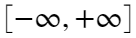along each dimension of the objective function. This is a fine default assumption for this particular simulation and in most other scenarios. If needed, you can change them via the following attributes,
pmpd.spec.domainLowerLimitVec = -1.e300 * ones(NDIM,1); % set the lower limits of the domain of the objective function to negative infinity (This is the default value)
pmpd.spec.domainUpperLimitVec = +1.e300 * ones(NDIM,1); % set the upper limits of the domain of the objective function to negative infinity (This is the default value)
The ParaDRAM sampler guarantees to not sample any points outside the hypercube defined by the above limits.

===============================
Note that none of the above specification settings were necessary, but is in general a good habit to define them prior to the simulation.
We now call the ParaDRAM sampler routine to run the sampling simulation. For the same of this simulation, we will also make the output files overwritable (before doing, The ParaDRAM sampler method takes only two arguments. We can call it like the following,
pmpd.spec.overwriteRequested = true; % overwrite the simulation output files if they already exist
pmpd.runSampler ( NDIM ... This is the number of dimensions of the objective function
, getLogFunc ... This is the objective function
);
• On Windows operating systems (OS), the sampler will print the realtime simulation progress information on your MATLAB prompt window.
• On macOS / Linux OS, the sampler will print the realtime simulation progress information on the Bash terminal from which you invoked the MATLAB executable to open the MATLAB application.

## The simulation output files

================================
Once the simulation is finished, the ParaDRAM routine generates 5 output files, each of which contains information about certain aspects of the simulation,
• mvn_serial_process_1_report.txt: This file contains reports about all aspects of the simulation, from the very beginning of the simulation to the very last step, including the postprocessing of the results and final sample refinement.
• mvn_serial_process_1_sample.txt: This file contains the final fully-refined decorrelated i.i.d. random sample from the objective function.
• mvn_serial_process_1_chain.txt: This file contains the Markov chain, generally in either binary or condensed (compact) ASCII format to improve the storage efficiency of the chain on your computer.
• mvn_serial_process_1_progress.txt: This file contains dynamic realtime information about the simulation progress. The frequency of the progress report to this file depends on the value of the simulation specification progressReportPeriod.
• mvn_serial_process_1_restart.txt: This file contains the information required for the restart functionality. By default, it is written as a binary file. When requested as an ASCII-formatted file, it also contains aditional information about the adaptive updates to the proposal distribution during the simulation.
outPath = fullfile(currentDir,"out");
outFileList = ["mvn_serial_process_1_report.txt", "mvn_serial_process_1_progress.txt", "mvn_serial_process_1_sample.txt", "mvn_serial_process_1_chain.txt", "mvn_serial_process_1_restart.txt"];
for file = outFileList(end:-1:1)
open(fullfile(outPath,file))
end

## General Guidelines on the ParaMonte visualization tools

===================================================================
In the following sections, we will discuss some of the postprocessing and visualization tools that automatically ship with the ParaMonte library. However, this is just the tip of the iceberg. For more detailed instructions on how to use the individual visualization tools of the ParaMonte library, visist the following MATLAB live scripts,
line / scatter plots
density plots
other plots

## Reading the simulation output sample file

==================================================
You may have noticed that, upon finishing the simulation, the sampler provides hints on how to postprocess the results. We can read the generated output sample, contained in the file suffixed with *_sample.txt via,
ParaDRAM - WARNING: The ParaDRAM input simulation specification pmpd.spec.outputDelimiter is not set. ParaDRAM - WARNING: This information is essential for successful reading of the requested sample file(s). ParaDRAM - WARNING: Proceeding with the default assumption of comma-delimited sample file contents... ParaDRAM - NOTE: 1 files detected matching the pattern: "D:\temp\libparamonte_MATLAB\out\mvn_serial*" ParaDRAM - NOTE: processing file: "D:\temp\libparamonte_MATLAB\out\mvn_serial_process_1_sample.txt" ParaDRAM - NOTE: reading the file contents... ParaDRAM - NOTE: done in 1.645700 seconds. ParaDRAM - NOTE: ndim = 4, count = 11869 ParaDRAM - NOTE: computing the sample correlation matrix... ParaDRAM - NOTE: creating the heatmap plot object from scratch... ParaDRAM - NOTE: done in 1.126500 seconds. ParaDRAM - NOTE: computing the sample covariance matrix... ParaDRAM - NOTE: creating the heatmap plot object from scratch... ParaDRAM - NOTE: done in 0.219380 seconds. ParaDRAM - NOTE: computing the sample autocorrelation... ParaDRAM - NOTE: creating the line plot object from scratch... ParaDRAM - NOTE: creating the scatter plot object from scratch... ParaDRAM - NOTE: creating the lineScatter plot object from scratch... ParaDRAM - NOTE: done in 1.283500 seconds. ParaDRAM - NOTE: adding the graphics tools... ParaDRAM - NOTE: creating the line plot object from scratch... ParaDRAM - NOTE: creating the scatter plot object from scratch... ParaDRAM - NOTE: creating the lineScatter plot object from scratch... ParaDRAM - NOTE: creating the line3 plot object from scratch... ParaDRAM - NOTE: creating the scatter3 plot object from scratch... ParaDRAM - NOTE: creating the lineScatter3 plot object from scratch... ParaDRAM - NOTE: creating the histogram plot object from scratch... ParaDRAM - NOTE: creating the histogram2 plot object from scratch... ParaDRAM - NOTE: creating the histfit plot object from scratch... ParaDRAM - NOTE: creating the contour plot object from scratch... ParaDRAM - NOTE: creating the contourf plot object from scratch... ParaDRAM - NOTE: creating the contour3 plot object from scratch... ParaDRAM - NOTE: creating the grid plot object from scratch... ParaDRAM - NOTE: The processed sample files are now stored in the newly-created component "pmpd.sampleList" of the ParaDRAM - NOTE: ParaDRAM object as a cell array. For example, to access the contents of the first (or the only) sample ParaDRAM - NOTE: file, try: ParaDRAM - NOTE: ParaDRAM - NOTE: pmpd.sampleList{1}.df ParaDRAM - NOTE: ParaDRAM - NOTE: To access the plotting tools, try: ParaDRAM - NOTE: ParaDRAM - NOTE: pmpd.sampleList{1}.plot.<PRESS TAB TO SEE THE LIST OF PLOTS> ParaDRAM - NOTE: ParaDRAM - NOTE: For example, ParaDRAM - NOTE: ParaDRAM - NOTE: pmpd.sampleList{1}.plot.line.make() % to make 2D line plots. ParaDRAM - NOTE: pmpd.sampleList{1}.plot.scatter.make() % to make 2D scatter plots. ParaDRAM - NOTE: pmpd.sampleList{1}.plot.lineScatter.make() % to make 2D line-scatter plots. ParaDRAM - NOTE: pmpd.sampleList{1}.plot.line3.make() % to make 3D line plots. ParaDRAM - NOTE: pmpd.sampleList{1}.plot.scatter3.make() % to make 3D scatter plots. ParaDRAM - NOTE: pmpd.sampleList{1}.plot.lineScatter3.make() % to make 3D line-scatter plots. ParaDRAM - NOTE: pmpd.sampleList{1}.plot.contour3.make() % to make 3D kernel-density contour plots. ParaDRAM - NOTE: pmpd.sampleList{1}.plot.contourf.make() % to make 2D kernel-density filled-contour plots. ParaDRAM - NOTE: pmpd.sampleList{1}.plot.contour.make() % to make 2D kernel-density plots. ParaDRAM - NOTE: pmpd.sampleList{1}.plot.histogram2.make() % to make 2D histograms. ParaDRAM - NOTE: pmpd.sampleList{1}.plot.histogram.make() % to make 1D histograms. ParaDRAM - NOTE: pmpd.sampleList{1}.plot.grid.make() % to make GridPlot ParaDRAM - NOTE: ParaDRAM - NOTE: To plot or inspect the variable autocorrelations or the correlation/covariance matrices, try: ParaDRAM - NOTE: ParaDRAM - NOTE: pmpd.sampleList{1}.stats.<PRESS TAB TO SEE THE LIST OF COMPONENTS> ParaDRAM - NOTE: ParaDRAM - NOTE: For more information and examples on the usage, visit: ParaDRAM - NOTE: ParaDRAM - NOTE: https://www.cdslab.org/paramonte
sample = pmpd.sampleList{1};
By default, the ParaDRAM sampler generates a highly refined decorrelated final sample, essentially refining the Markov chain for as long as there is any residual auto-correlation in any of the individual variables of the Markov chain that has been sampled. This is an extremely strong and conservative refinement strategy (and it is so for a good reason: to ensure independent and identically distributed (i.i.d.) samples from the objective function). However, this extreme refinement can be mitigated and controlled by the setting the following ParaDRAM simulation specification variable prior to the simulation run,
pmpd.spec.sampleRefinementCount = 1; % refine each of the compact and verbose (Markov) chains only once.
pmpd.spec.helpme("sampleRefinementCount")
Rerun the simulation with this specification set and you will notice an increase in the final sample size by a few factors. Note that the above specification has to be set before running the simulation in the above, if you really want to use it.

#### Visualizing the refined sample

To quickly visualize the generated sample as a histogram, try,
sample.plot.histogram.make()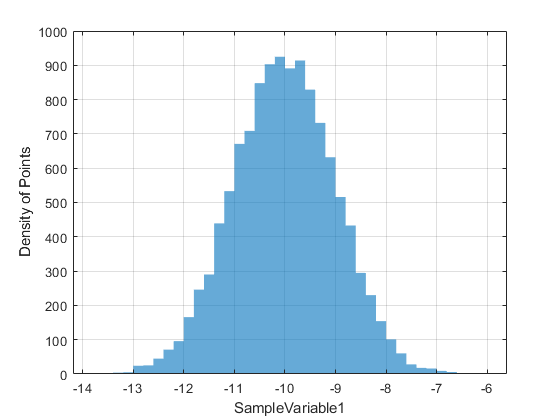To plot all of the sampled variables, try,
sample.plot.histogram.reset(); % reset the plot properties to the default
ParaDRAM - NOTE: resetting the properties of the histogram plot...
sample.df.Properties.VariableNames
ans = 1×5 cell array {'SampleLogFunc'} {'SampleVariable1'} {'SampleVariable2'} {'SampleVariable3'} {'SampleVariable4'}
sample.plot.histogram
ans =
DensityPlot with properties: xcolumns: "SampleVariable1" dfref: [11869×5 table] figure: [1×1 struct] currentFig: [1×1 struct] rows: {} axes: [1×1 struct] histogram: [1×1 struct] target: [1×1 Target_class] legend: [1×1 struct]
sample.plot.histogram.xcolumns = sample.df.Properties.VariableNames(2:end);
sample.plot.histogram.legend.kws.location = "northeast";
sample.plot.histogram.legend.enabled = true;
sample.plot.histogram.make();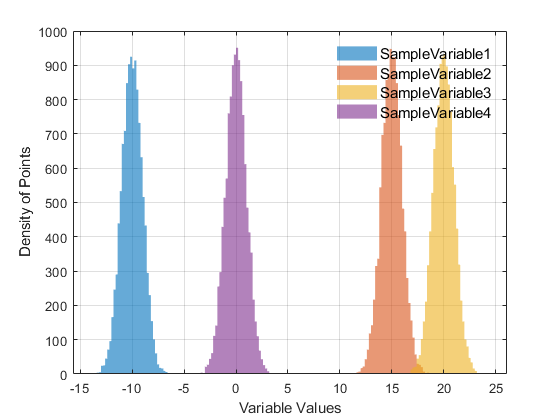Or, we could also used the column indices of the variabels, instead of the column names,
sample.plot.histogram.reset() % reset the plot properties to the default
ParaDRAM - NOTE: resetting the properties of the histogram plot...
sample.plot.histogram.legend.enabled = false; % let's also remove the legends
sample.plot.histogram.make("xcolumns",[2,3,4,5]); % the column indices of all sampled variables in the output sample file.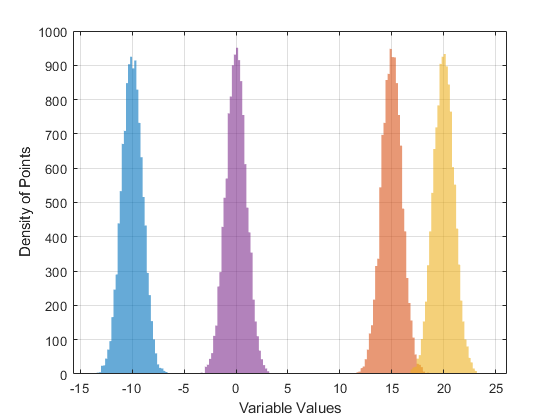We can also rebuild the histogram object from scratch, which includes reading again the input data used in the plotting from the dataFrame, by specifying the input parameter "hard",
sample.plot.histogram.reset("hard")
ParaDRAM - NOTE: creating the histogram plot object from scratch...
Make a trace plot of the refined sample,
sample.plot.line.reset();
ParaDRAM - NOTE: resetting the properties of the line plot...
sample.plot.line.colormap.values = "autumn"; % let's request autumn color-mapping
sample.plot.line.make();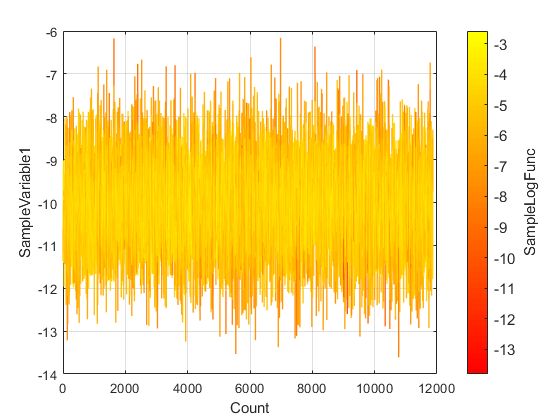We can simply take the axis logarithmic scale,
sample.plot.line.axes.kws.xscale = "log";
sample.plot.line.make();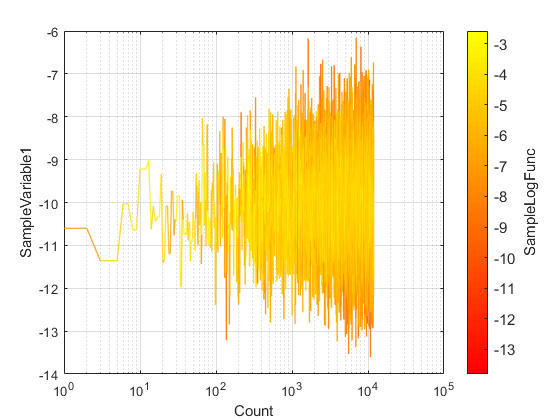or, we could do it via,
set(gca,'xscale','log','yscale','linear');We can also include more variables in a single trace plot, for example, the the first, second, and the fourth dimensions of the objective function,
sample.plot.line.reset(); % reset all settings including colormap to the default values.
ParaDRAM - NOTE: resetting the properties of the line plot...
sample.plot.line.make("ycolumns",[2,3,5]); % these are the column numbers in the dataFrame sample.df
set(gca,'xscale','log','yscale','linear');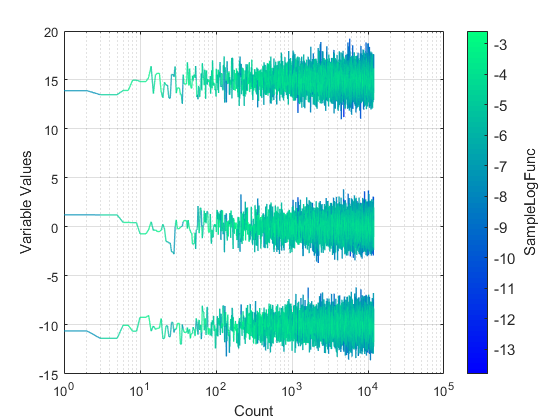By default, the color of the line in the trace-plot will represent the value returned by getLogFunc() at the given sampled point.
sample.plot.line.ccolumns
ans = "SampleLogFunc"
To turn the color mapping off, you can instead try,
sample.plot.line.reset(); % reset to the default settings
ParaDRAM - NOTE: resetting the properties of the line plot...
sample.plot.line.colormap.enabled = false; % disable varying-color lines
sample.plot.line.legend.enabled = true; % display legend
sample.plot.line.legend.kws.location = "best";
sample.plot.line.make("ycolumns",3:5); % these are the column numbers in the dataFrame sample.df
set(gca,'xscale','log','yscale','linear');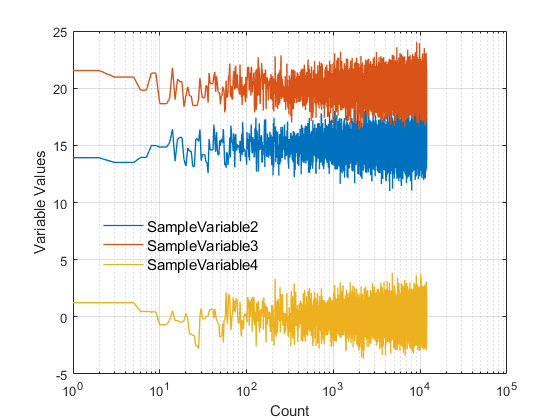We can also change the properties of the lines as we wish,
sample.plot.line.reset();
ParaDRAM - NOTE: resetting the properties of the line plot...
sample.plot.line.colormap.enabled = false;
sample.plot.line.legend.enabled = true; % display legend
sample.plot.line.plot.kws.linewidth = 1.0;
sample.plot.line.plot.kws.linestyle = ":"; % we can add any new proprty to plot_kws, that is an input argument to MATLAB's plot().
sample.plot.line.make("ycolumns",["SampleLogFunc",3:5]); % we can mix column names with column numbers
set(gca,'xscale','log','yscale','linear');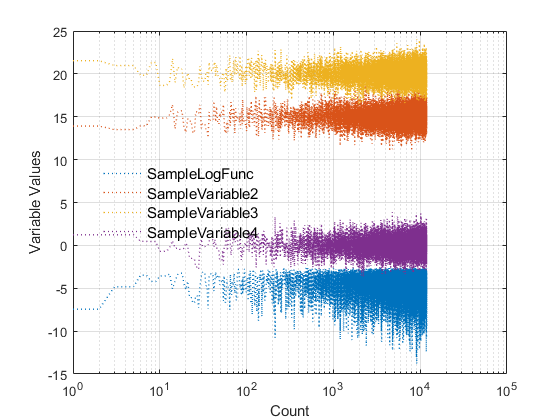To make a scatter trace-plot of the sampled points, try,
sample.plot.scatter.make();